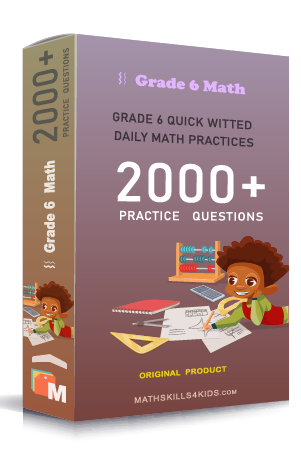# Grade 6 Geometric measurements worksheets with solutions

Subject
Math

Resource Type
Worksheets, Printables, Homeschool

Standards
CCSS 6.G.A.1 | CCSS 6.G.A.2 | CCSS 6.G.A.3 | CCSS 6.G.A.4

Format
PDF (25.8 MB | 48 pages → Exercises + Solutions)

• ### -15% OFF Over \$100

Promo Code: TOPLA

After Purchase

\$4.85

This package allows you to practice the following skills

Finding perimeters of figures

Finding areas of rectangles and squares

Finding areas of triangles

Finding areas of parallelograms and trapezoids

Finding areas of compound figures

Finding the area between two rectangles

Finding the area between two triangles

Understanding the relationship between perimeters and surfaces of rectangles

Comparing the areas and surfaces of two figures

Circles: calculate area, radius, circumference, and diameter

Circles word problems

Calculate the area between two circles

Calculate the volume of cubes and rectangular prisms

Solving volume of cubes and rectangular prisms word problems

Calculate the surface area of cubes and rectangular prisms

This product is a part of the Mega Pack
* Grade 6 quick-witted daily math practices *Calculate the volume of triangular prisms

Calculate the surface area of triangular prisms

Calculate the surface area of pyramids

Calculate the volume and surface area of cylinders

Understanding the relationship between the volume and surface area of cubes

Semicircles: calculate area, radius, and diameter

Quater circles: calculate area, radius, and diameter

Calculate the area of compound figures with triangles and rectangles